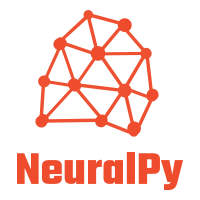# neuralpy-torch

Showing:

0

79

4mos ago

4

## Package

5

MIT

### CategoriesA Keras like deep learning library works on top of PyTorch## Introduction

NeuralPy is a High-Level Keras like deep learning library that works on top of PyTorch written in pure Python. NeuralPy can be used to develop state-of-the-art deep learning models in a few lines of code. It provides a Keras like simple yet powerful interface to build and train models.

Here are some highlights of NeuralPy

• Provides an easy interface that is suitable for fast prototyping, learning, and research
• Can run on both CPU and GPU
• Works on top of PyTorch
• Cross-Compatible with PyTorch models

## PyTorch

PyTorch is an open-source machine learning framework that accelerates the path from research prototyping to production deployment developed by Facebook runs on both CPU and GPU.

According to Wikipedia,

PyTorch is an open-source machine learning library based on the Torch library, used for applications such as computer vision and natural language processing, primarily developed by Facebook's AI Research lab (FAIR). It is free and open-source software released under the Modified BSD license.

NeuralPy is a high-level library that works on top of PyTorch. As it works on top of PyTorch, NerualPy supports both CPU and GPU and can perform numerical operations very efficiently.

## Install

To install NeuralPy, open terminal window type the following command:

``````pip install neuralpy-torch
``````

If you have multiple versions of it, then you might need to use pip3.

``````pip3 install neuralpy-torch
//or
python3 -m pip install neuralpy-torch
``````

NeuralPy requires Pytorch and Numpy, first install those

Check the documentation for Installation related information

## Dependencies

The only dependencies of NeuralPy are Pytorch (used as backend) and Numpy.

## Get Started

Let's create a linear regression model in 100 seconds.

### Importing the dependencies

``````import numpy as np

from neuralpy.models import Sequential
from neuralpy.layers.linear import Dense
from neuralpy.loss_functions import MSELoss
``````

### Making some random data

``````# Random seed for numpy
np.random.seed(1969)

# Generating the data
X_train = np.random.rand(100, 1) * 10
y_train = X_train + 5 *np.random.rand(100, 1)

X_validation = np.random.rand(100, 1) * 10
y_validation = X_validation + 5 * np.random.rand(100, 1)

X_test = np.random.rand(10, 1) * 10
y_test = X_test + 5 * np.random.rand(10, 1)
``````

### Making the model

``````# Making the model
model = Sequential()

# Building the model
model.build()

# Compiling the model

# Printing model summary
model.summary()
``````

### Training the model

``````model.fit(train_data=(X_train, y_train), validation_data=(X_validation, y_validation), epochs=300, batch_size=4)
``````

### Predicting using the trained model

``````model.predict(predict_data=X_test, batch_size=4)
``````

## Documentation

The documentation for NeuralPy is available at https://www.neuralpy.xyz/

## Examples

Several example projects in NeuralPy are available at https://github.com/imdeepmind/NeuralPy-Examples. Please check the above link.

## Blogs and Tutorials

Following are some links to official blogs and tutorials:

## Support

If you are facing any issues using NeuralPy, then please raise an issue on GitHub or contact with me.

Alternatively, you can join the official NeuralPy discord server. Click here to join.

## Contributing

Feel free to contribute to this project. If you need some help to get started, then reach me or open a GitHub issue. Check the CONTRIBUTING.MD file for more information and guidelines.

MIT

## Rate & Review

Great Documentation0
Easy to Use0
Performant0
Highly Customizable0
Bleeding Edge0
Responsive Maintainers0
Poor Documentation0
Hard to Use0
Slow0
Buggy0
Abandoned0
Unwelcoming Community0
100No reviews found
Be the first to rate

## AlternativesNo alternatives found

## TutorialsNo tutorials found